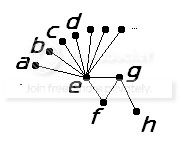# Graphs with few induced subgraphs

Which graphs have the property that the number of $i$-vertex induced subgraphs is at most $i$ for some $i < n/2$ (where $n$ is the number of vertices)?

To avoid cases I am not interested in, I want the graph to be connected and non-bipartite and its complement to have the same properties.

Of course every graph has this property for $i=2$, and every triangle-free graph (or complement of one) for $i=3$. What about larger values of $i$?

Edit. More background information on the problem can be found here.

• Welcome to MathOverflow! – Douglas S. Stones Aug 5 '11 at 10:11
• Should this be tagged open-problem? – Emil Aug 6 '11 at 11:42

## 3 Answers

Here's an example $G$ for all $i \geq 8$ and $n \geq 2i+1$ that contains only $8$ induced subgraphs (up to isomorphism).

If we choose $i$ vertices from the top row, we obtain $\overline{K_i}$.

If we choose $i-1$ vertices from the top row, we obtain $K_{1,i-1}$ or $\overline{K_i}$.

If we choose $i-2$ vertices from the top row, we obtain $\overline{K_i}$ or $\overline{K_{i-2}} \cup K_2$ or $K_{1,i-2} \cup K_1$ or $K_{1,i-1}$.

If we choose $i-3$ vertices from the top row, we obtain the subgraphs induced by $i-3 \text{ vertices from a,b,c,d,... } \cup \{e,g,h\}$ or $i-3 \text{ vertices from a,b,c,d,... } \cup \{e,f,g\}$ or $K_{1,i-2} \cup K_1$ or $\overline{K_{i-3}} \cup P_3$ (path with three vertices).

If we choose $i-4$ vertices from the top row, we obtain the graph induced by $i-4 \text{ vertices from a,b,c,d,... } \cup \{e,f,g,h\}$.

Since we must choose at least $i-4$ vertices from the top row, in total, that's 8 isomorphism classes of graphs. We can see that $G$ and its complement both contain a triangle (and are therefore not bipartite) and are connected (each vertex is not adjacent to either h or a).Generalising this technique, we can replace {e,f,g,h} by any non-bipartite connected subgraph of diameter at least 2. In this case, there would exist some I, N such that for all i>=I and n>=max(N,2i+1) which would satisfy the required conditions.

• The vertex $e$ seems isolated in the complement... – M P Aug 5 '11 at 12:11
• Ouch! Hmm... that's a problem! – Douglas S. Stones Aug 5 '11 at 12:12
• Hmm... I think the idea can be salvaged for larger values of i; e.g. be attaching a leaf to g. – Douglas S. Stones Aug 5 '11 at 12:19
• Right, I think it's fixed now. Wow, that was a bookkeeping nightmare. – Douglas S. Stones Aug 5 '11 at 12:48

Thanks, Doug. But, of course, I am not satisfied with this answer! I would like the graph to be vertex-transitive, and edge-transitive. I already know that its diameter is at most 4 (in my application).

I will come a bit cleaner about the application. This arises in a question of Joao Araujo about semigroup theory, which reduces to the following: Which groups are $(i,i+1)$-homogeneous but not $i$-homogeneous? (The condition $(i,i+1)$-homogeneous means that given any $i$-set $I$ and $j$-set $J$, there is an element of the group mapping $I$ to a subset of $J$.

Assume that $2i+1\le n$. Such a group is easily seen to be primitive and have at most three orbits on $2$-sets. If it is $2$-homogeneous, then it must be $2$-transitive, and then one has the possibility of using the classification of such groups.

It is easy to see that such a group has at most $i$ orbits on $i$-sets; so if it is not $2$-homogeneous, then all the symmetrised orbital graphs have the property of my original question.

By the way, five examples of groups with this property are known: the cyclic and dihedral groups of degree 5, and the affine groups $\mathrm{AGL}(1,7)$, $\mathrm{ASL}(2,3)$ and $\mathrm{AGL}(2,3)$ (with $n=7,9,9$ for the last three).

This is a pretty interesting question. Douglas S. Stones' answer provides an example that works (but has diameter 3). On the other hand, large diameter forces many induced subgraphs for all small values of $i$.

Lemma. Let $G$ be a graph with diameter $d \geq 8$. Then for all $4 \leq i \leq d/2$, the number of induced subgraphs of $G$ with $i$ vertices is more than $i$.

Proof. Let $P$ be an induced path of $G$ with $d$ vertices. Let $F_i$ be the set of all forests on $i$ vertices with maximum degree 2. Observe that $P$ contains all graphs in $F_i$ for all $i \leq d/2$ as induced subgraphs. Since $F_i > i$ for all $i \geq 4$, we are done.

The property seems harder to satisfy for larger values of $i$ which leads us to the following (updated) rash conjecture.

Rash Conjecture. Let $G$ be a connected, non-bipartite graph on $n$ vertices whose complement is also connected and non-bipartite. If $G$ has at most $i$ induced $i$-subgraphs for some $3 < i < n/2$, then $G$ has diameter at most 7.

• Note: 4 is not less than 5/2. – Douglas S. Stones Aug 5 '11 at 11:29
• Indeed, edited accordingly. – Tony Huynh Aug 5 '11 at 11:36
• Only odd cycle of length 9 works. Otherwise you get (a) null graph, (b) P_2, (c) P_3, (d) P_4 and (e) P_2 \cup P_2. [where P_i = path with i vertices]. With a 9-cycle, you can't get an induced null graph. – Douglas S. Stones Aug 5 '11 at 11:49
• Ah, I missed $P_3$ for some reason. But I also don't think $C_9$ works either since it does contain the null graph as an induced subgraph. – Tony Huynh Aug 5 '11 at 11:57
• I have described the background to the problem in a little more detail <a href="cameroncounts.wordpress.com/2011/08/05/mathoverflow/…>. – cameroncounts Aug 8 '11 at 9:04